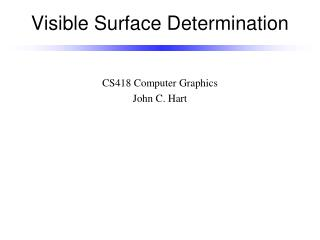Download PresentationVisible Surface Determination

# Visible Surface Determination - PowerPoint PPT PresentationDownload Presentation## Visible Surface Determination

- - - - - - - - - - - - - - - - - - - - - - - - - - - E N D - - - - - - - - - - - - - - - - - - - - - - - - - - -
##### Presentation Transcript

1. Visible Surface Determination CS418 Computer Graphics John C. Hart

2. Painter’s Algorithm • Display polygons inback-to-front order • Sort polygons by z-value • Which vertex? • O(n log n) • Problems… -z

3. Quadtree Algorithm • Sort polygons • Subdivide screen until each region contains one or zero edges • Invented by John Warnock in 1969

4. Quadtree Algorithm • Sort polygons • Subdivide screen until each region contains one or zero edges • Invented by John Warnock in 1969

5. Quadtree Algorithm • Sort polygons • Subdivide screen until each region contains one or zero edges • Invented by John Warnock in 1969

6. Quadtree Algorithm • Sort polygons • Subdivide screen until each region contains one or zero edges • Invented by John Warnock in 1969

7. Quadtree Algorithm • Sort polygons • Subdivide screen until each region contains one or zero edges • Invented by John Warnock in 1969

8. Z-Buffer -far -far -far -far Key Observation: Each pixel displays color of only one triangle, ignores everything behind it • Don’t need to sort triangles, just find for each pixel the closest triangle • Z-buffer: one fixed or floating point value per pixel • Algorithm: For each rasterized fragment (x,y) If z > zbuffer(x,y) then framebuffer(x,y) = fragment color zbuffer(x,y) = z -far -far -far -far -far -far -far -far zbuffer framebuffer

9. Z-Buffer -far -.1 -.2 -.3 Key Observation: Each pixel displays color of only one triangle, ignores everything behind it • Don’t need to sort triangles, just find for each pixel the closest triangle • Z-buffer: one fixed or floating point value per pixel • Algorithm: For each rasterized fragment (x,y) If z > zbuffer(x,y) then framebuffer(x,y) = fragment color zbuffer(x,y) = z -.4 -.5 -.6 -.7 -.8 -far -far -far zbuffer framebuffer

10. Z-Buffer -far -.1 -.2 -.3 Key Observation: Each pixel displays color of only one triangle, ignores everything behind it • Don’t need to sort triangles, just find for each pixel the closest triangle • Z-buffer: one fixed or floating point value per pixel • Algorithm: For each rasterized fragment (x,y) If z > zbuffer(x,y) then framebuffer(x,y) = fragment color zbuffer(x,y) = z -.4 -.3 -.1 -.7 -.8 -far -far -far zbuffer framebuffer

11. Z-Buffer -far -.1 -.2 -.3 • Get fragment z-values by interpolating z-values at vertices during rasterization • Perspective projection destroysz-values, setting them all to –d • Need a perspective distortion that preserves at least the ordering ofz-values -.4 -.3 -.1 -.7 -.8 -far -far -far zbuffer framebuffer

12. Normalized View Volume glFrustum(left,right,bottom,top,near,far) y y (-1,1,1) (left,top,-near) 1 z z (0,0,-far) y x x -1 (right,bottom,-near) -1 x 1 z 1 -1 ScreenCoords ClipCoords ViewingCoords WorldCoords ModelCoords

13. Perspective Projection screen y yview yclip -z zview d

14. Perspective Distortion screen y yview yclip -z zview 1

15. Distorted z-Values z1 z2 -z if z1 > z2 then -a – b/z1 > -a – b/z2 1/-z curve -a – b/z1 -a – b/z2 -a -a – b/z

16. Normalized Perspective Distortion y y (-1,1,1) (left,top,-near) 1 z z (0,0,-far) y x x -1 (right,bottom,-near) -1 x 1 z 1 -1

17. Hierarchical Z-Buffer -.2 -.3 -.5 -far -.3 • Invented by Ned Green in 1994 • Creates a MIP-map of the z-buffer • z-value equal to farthest z-value of its four children • Before rasterizing a triangle… • Check z-value of its nearest vertex against z-value of the smallest quadtree cell containing the triangle • If z-test fails, then the entire triangle is hidden and need not be rasterized • Works best when displayingfront-to-back -.4 -.5 -.5 -.6 -.7 -far -.7 -.8 -far -far hierarchical zbuffer framebuffer